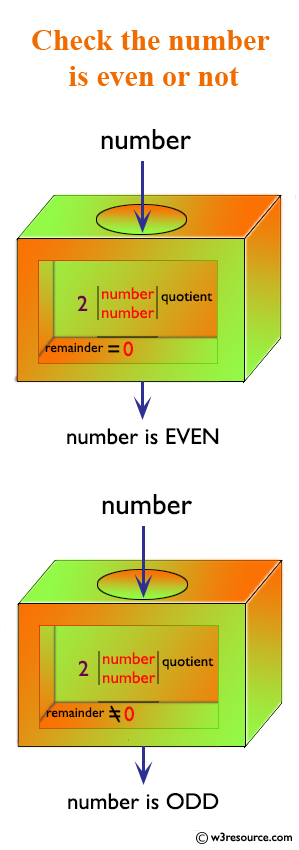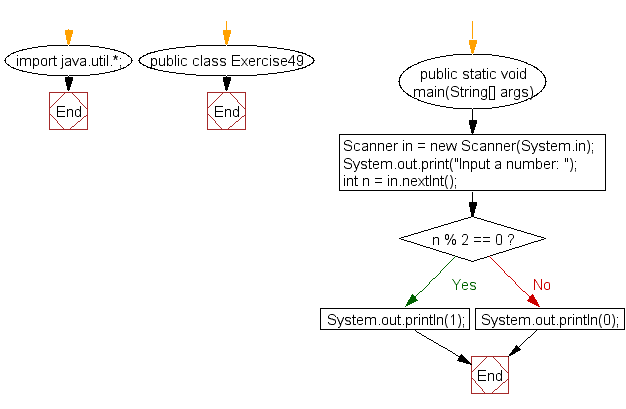﻿ Java: Accept a number and check the number is even or not# Java Exercises: Accept a number and check the number is even or not

## Java Basic: Exercise-49 with Solution

Write a Java program to accept a number and check the number is even or not. Prints 1 if the number is even or 0 if the number is odd.

Pictorial Presentation:Sample Solution:

Java Code:

``````import java.util.*;
public class Exercise49 {
public static void main(String[] args){
Scanner in = new Scanner(System.in);
System.out.print("Input a number: ");
int n = in.nextInt();
if (n % 2 == 0) {
System.out.println(1);
}
else {
System.out.println(0);
}
}
}
```
```

Sample Output:

```Input a number: 20
1
```

Flowchart:Java Code Editor:

What is the difficulty level of this exercise?

Test your Programming skills with w3resource's quiz.

﻿

## Java: Tips of the Day

System.currentTimeMillis vs System.nanoTime

In Java, there are two standard ways of conducting time-operations and it is not always clear which one should be chosen.

The method System.currentTimeMillis() returns the current number of milliseconds since the beginning of the Unix era in the format Long. Its accuracy ranges from 1 to 15 thousandths of a second, depending on the system.

`long startTime = System.currentTimeMillis();long estimatedTime = System.currentTimeMillis() - startTime;`

The method System.nanoTime has an accuracy of up to one-millionth of a second (nanoseconds) and returns the current value of the most accurate available system timer.

```long startTime = System.nanoTime();long estimatedTime = System.nanoTime() - startTime;
```

Thus, it is System.currentTimeMillisexcellent for displaying and synchronizing absolute time, and System.nanoTime for measuring relative intervals.

Ref: https://bit.ly/2W4V97w